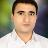# Gas networks simulation from disaggregation of low frequency nodal gas consumption

Published: 24 Dec 2016 | Version 1 | DOI: 10.17632/fyz68byfxs.1
Contributor(s):Engineering
Natural Gas Networks

## Description of this data

Dear Researcher,

Thank you for using these codes and datasets. I explain how Time Series Disaggregation (TSD) methods introduced in my paper "Gas networks simulation from disaggregation of low frequency nodal gas consumption" published in Energy, works. All datasets mentioned in the paper accompanied with codes of TSD methods are included.
If there is any question feel free to contact me at:

Regards,

Opening the folder "Gas networks simulation from disaggregation of low frequency nodal gas consumption" you'll find some Codes, Functions, and Datasets which are described as follows.

Codes and Functions:

1. "gnetplot": This function plots gas networks.
2. "TSD-UnrelatedTimeSeries": This code disaggregates unrelated time series.
3. "TSD-RelatedTimeSeries": This code disaggregates related time series.
4. "NetworkSolution": This code loads the network properties and nodal consumption and then solves the network governing equations and computes nodal pressure of the network for each day.

Datasets:

1. "EX1": This dataset includes one time series (It is shown in Fig. 3 of the paper.).
2. "EX2": This dataset includes six related time series (It is shown in Fig. 4 of the paper.).
3. "EX3": This dataset includes 140 related time series.
4. "EX3Solved": This dataset includes the dataset "EX3" and its solution. In fact these data are results of applying "TSD-RelatedTimeSeries" to the data "EX3".
5. "GasNetworkProperties": This datasets includes properties of the gas network studied in the paper and shown in Fig. 1.
6. "Results": This file includes some of the results and graphs given in the paper.

Guidelines for the codes:
Open one of the codes "TSD-UnrelatedTimeSeries", "TSD-RelatedTimeSeries", and "NetworkSolution" using MATLAB. Guidelines for working with each code are as follows.

1. "TSD-UnrelatedTimeSeries":
This code disaggregates single or multiple unrelated time series. Line 15 of the code loads the data to be disaggregated. You just need to type name of the dataset after "load" to load the data. The dataset EX1 is for this code. You need to press Ctrl+Enter to run the code. For your own dataset, arrange the data as the sample dataset EX1. According to the theory given in the paper, the data should include matrix of independent variables and matrix of unrelated time series where is number of high frequency data, is number of low frequency data, is number of independent variables, and is number of unrelated time series. The vector is the original high frequency data from which is constructed by aggregation. After disaggregation, the resulted high frequency data can be compared with its original version to verify accuracy of the proposed TSD method.

## Steps to reproduce

1. "TSD-RelatedTimeSeries":
This code disaggregates related time series. Line 19 of the code loads the data to be disaggregated. You just need to type name of the dataset after "load" to load the data. The datasets EX2 and EX3 are for this code. You need to press Ctrl+Enter to run the code. For your own dataset, arrange the data as the sample dataset EX2 or EX3. According to the theory given in the paper, the data should include matrix of independent variables , matrix of related time series , and the vector , where is number of high frequency data, is number of low frequency data, is number of independent variables, and is number of related time series. In each dataset, the matrix is the original high frequency data from which and are constructed by aggregation. After disaggregation, the resulted high frequency data can be compared with its original version to verify accuracy of the proposed TSD method.

2. "GasNetworkProperties":
This code solves gas networks provided that the network properties are available. Line 10 loads the network properties and line 59 loads nodal gas consumption of each 140 nodes of the network. The dataset EX3 which includes 140 related low frequency (monthly) time series is for this network where each time series is low frequency gas consumption of one node. These data includes 1200 high frequency (daily) data and 30 low frequency (monthly) data. The vector is high frequency (daily) gas consumption of the entire network recorded by the CGS gas meter. Applying "TSD-RelatedTimeSeries" to EX3 disaggregates these data to high frequency (daily) gas consumption of the nodes which is stored as "EX3Solved". "GasNetworkProperties" loads "EX3Solved" and from these data reads daily gas consumption of each node which is to solve the network governing equations and compute nodal pressure.

For details, please note the "Read Me" file in the uploaded folder named "Gas networks simulation from disaggregation of low frequency nodal gas consumption".

This data is associated with the following publication:

Gas networks simulation from disaggregation of low frequency nodal gas consumption

• ### Version 1

2016-12-24

Published: 2016-12-24

DOI: 10.17632/fyz68byfxs.1

### Cite this dataset

Askari Lasaki, Salar (2016), “Gas networks simulation from disaggregation of low frequency nodal gas consumption”, Mendeley Data, v1 http://dx.doi.org/10.17632/fyz68byfxs.1

Views: 547